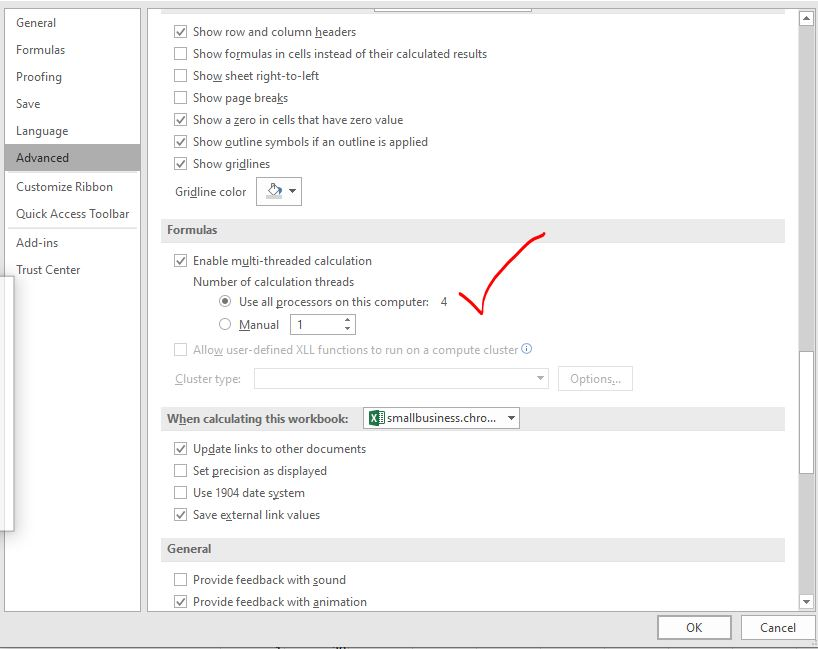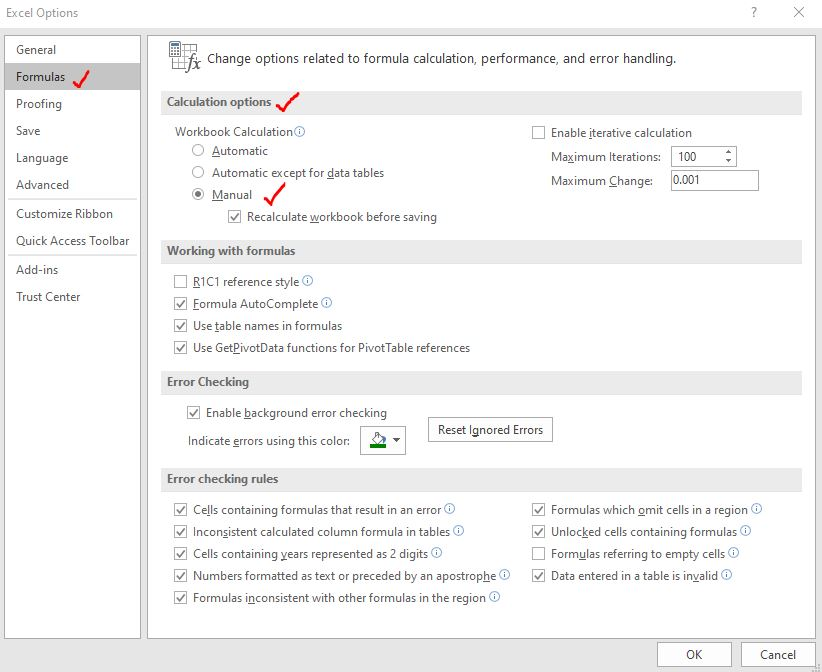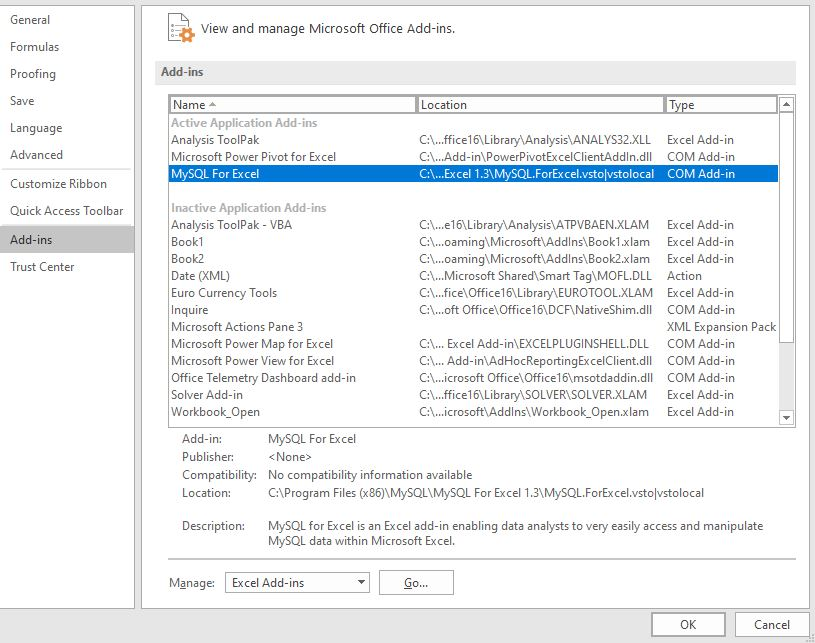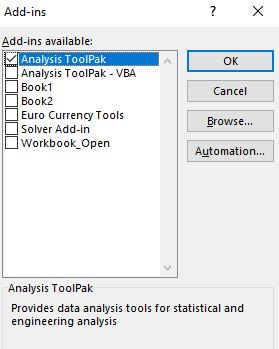# 13 Methods of How to Speed Up ExcelExcel is fast enough to calculate 6.6 million formulas in 1 second in Ideal conditions with normal configuration PC. But sometimes we observe excel files doing calculation slower than snails. There are many reasons behind this slower performance. If we can Identify them, we can make our formulas calculate faster. In this article, we will learn how can we make our excel files calculate faster.

These are the methods that can make your excel calculate faster.

1 : Reduce the complexity and number of formulas

I know I said that excel can calculate 6.6 million formulas in one second. But in Ideal conditions. Your computer is not only using Excel program right. It has other tasks running.

Excel does not only have formulas. It has data, tables, charts, add inns, etc. All these increase the burden on the processor. And when you have thousands  of complex formulas running, it slows down the processor.

So, if it is possible, reduce the number of the formulas and complexity of formulas.

2. Use all the processors available for Excel.

Some times your computer is busy in processing other threads. Making all the processors available for excel will increase the speed of Excel significantly. To do so follow these steps:

Go to file --> Options --> Advanced. Here scroll down and find Formulas Section. Select the "Use all the processors in this computer: 4". This will allow excel to use all the processors available on the system. It will make the calculations fast.Now excel calculating using 4 processors on my system. It can be more or less on your system.

3: Avoid use of  Volatile Functions

The volatile functions like NOW(), OFFSET(), RAND(), etc. recalculate every-time a change is made in Excel file. This increases the burden on the processors. When you have a large number of formulas in the workbook and you make any change in the excel file, the whole file starts to recalculate, even if you don't want it. This can take too much time and even make Excel crash. So if you have a large number of formulas in the excel file make sure they are not volatile functions.

Some commonly used volatile functions are, OFFSET, RAND, NOW, TODAY, RANDBETWEEN, INDIRECT, INFO, CELL.

4: Switch to Manual Calculation in Excel

If you got have large number of complex formulas with or without volatile functions, set the workbook calculation to manual.

This does not mean that you have to do manual calculations. It just mean that you will tell excel when to recalculate the workbook.

By default, Excel is set to automatic calculations. Due to automatic calculations you can see the change in result of a formula when you change the source data, instantly. This slows the Excel speed.

But if you use manual calculation, the change will not reflect instantly. Once you have done all the task in the sheet, you can use F9 key to tell excel to recalculate the workbook.  This will save a lot of resource and time.

To switch to manual calculations, go to File --> Options --> Formulas. You will have a number of options available. Find calculation option and select the manual calculation and hit the OK button.5: Do not use Data Tables for Large Data Set

The data tables give a lot functionality that is really helpful. But these functionalities are costly in terms of resources. If you have a large set data than you should avoid using excel data tables. They use a lot of computer resources and can slow down the performance of Excel file. So, if it is not necessary, avoid using Data table for faster calculation of formulas. This will increase formula calculation speed.

6: Replace the Static Formulas with Values:

If you have a large set of calculations and some of the formulas does not change the results, it is better to replace the with values. For example, if you are using VLOOKUP function to import or merge tables for one time, it is better to replace the formula with values.

To do so, copy the result range. Right-Click on the range and go to paste special. Here select Value and hit the OK button. You can read about pasting value here.

7: Disabling the Excel Add ins

The Excel add ins are additional functionalities we add to the tool. They may not needed every time. If you can disable them, you can save a lot of resource on your system. This will help you increase the formula calculation speed.

To disable the add ins go to File --> Options --> Add Ins. Select the add ins and click on Go button.This will open the add active add ins. Uncheck the add ins that you don't need. Hit OK button.This will disable the add ins.

8: Avoid Unnecessary Conditional Formatting in workbook.

The conditional formatting takes a lot of calculation time so it is better to only use them where it is necessary. Do not use the conditional formatting the large data set.

9: Remove unnecessary pivot tables

The pivot tables are great tool for quickly summarizing a large set of data. But if you need the pivot table only for one time, use and delete them. You can keep the value pasted pivot report. If you want to have dynamic data, use formulas.

10: Replace Formulas with VBA Macros

There's nothing you can't do using Macros. Sometime it is even more handy and easy to do calculations in VBA than directly on worksheet. You can use the worksheetfunctions object to access the worksheet function VBA. Use them to do large number of calculation and paste value using VBA. This will increase the speed of Excel calculations exponentially.

11: Delete Unused Ranges and Sheets

It is common to use the spare sheets and ranges while working. But you might not know that excel remembers in which ranges you have worked and keeps it into used ranges memory. It is smart delete such sheets and ranges from workbook, so that excel does not uses them any more.

12: Save Excel File as Excel Binary File

If your excel file does not requires to interact with other tools than you should save the excel file as Excel Binary File. The extension of an Excel binary file is .xlsb. This does removes any functionality from excel. Your file just won't be able to interact with other tools.

13: Consider Using Database tools for storing data.

Most of the time Excel formula calculations slows down due to large amount of data in the workbook. So if you can save the source data in some other database tool or CSV file and use excel for only calculation than you can increase the speed of formula calculations. You can use power pivot or power query to import data temporarily for analyzing data.

So yeah guys, these are the ways to increase the speed of formula calculation in Excel. If you do all these things you will surely increase the calculation speed and excel will not stop or crash while performing calculations.

I hope it was explanatory and helpful. If you have any doubt or special scenario, please let me know in the comments section below. I will be happy to hear from you. Till than keep Excelling.

Related Articles:

Center Excel Sheet Horizontally and Vertically on Excel Page : Microsoft Excel allows you to align worksheet on a page, you can change margins, specify custom margins, or center the worksheet horizontally or vertically on the page. Page margins are the blank spaces between the worksheet data and the edges of the printed page

Split a Cell Diagonally in Microsoft Excel 2016 : To split cells diagonally we use the cell formatting and insert a diagonally dividing line into the cell. This separates the cells diagonally visually.

How do I Insert a Check Mark in Excel 2016 : To insert a checkmark in Excel Cell we use the symbols in Excel. Set the fonts to wingdings and use the formula Char(252) to get the symbol of a check mark.

How to disable Scroll Lock in Excel : Arrow keys in excel move your cell up, down, Left & Right. But this feature is only applicable when Scroll Lock in Excel is disabled.

Scroll Lock in Excel is used to scroll up, down, left & right your worksheet not the cell. So this article will help you how to check scroll lock status and how to disable it?

What to do If Excel Break Links Not Working : When we work with several excel files and use formula to get the work done, we intentionally or unintentionally create links between different files. Normal formula links can be easily broken by using break links option.

Popular Articles:

50 Excel Shortcuts to Increase Your Productivity | Get faster at your task. These 50 shortcuts will make you work even faster on Excel.

How to use Excel VLOOKUP Function| This is one of the most used and popular functions of excel that is used to lookup value from different ranges and sheets.

How to use the Excel COUNTIF Function| Count values with conditions using this amazing function. You don't need to filter your data to count specific value. Countif function is essential to prepare your dashboard.

How to Use SUMIF Function in Excel | This is another dashboard essential function. This helps you sum up values on specific conditions.

Terms and Conditions of use

The applications/code on this site are distributed as is and without warranties or liability. In no event shall the owner of the copyrights, or the authors of the applications/code be liable for any loss of profit, any problems or any damage resulting from the use or evaluation of the applications/code.